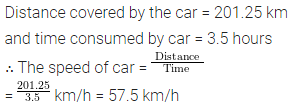# ML Aggarwal Class 6 Solutions for ICSE Maths Chapter 8 Ratio and Proportion Ex 8.5

## ML Aggarwal Class 6 Solutions for ICSE Maths Chapter 8 Ratio and Proportion Ex 8.5

Question 1.
The speed of a car is $$105 \frac{1}{5}$$ km/h, find the distance covered by it in $$3 \frac{3}{5}$$ hours.
Solution:Question 2.
If the speed of a car is 50.4 km/h, find the distance covered in 3.6 hours.
Solution:Question 3.
If a car covers a distance of 201.25 km in 3.5 hours, find the speed of the car.
Solution: